Courses

# Interconnected Synchronous Generators Notes | EduRev

## Electrical Engineering (EE) : Interconnected Synchronous Generators Notes | EduRev

The document Interconnected Synchronous Generators Notes | EduRev is a part of the Electrical Engineering (EE) Course Electrical Machines.
All you need of Electrical Engineering (EE) at this link: Electrical Engineering (EE)

Interconnected Synchronous Generators

The study of interconnection of several synchronous generators is important because of the following main reasons:

1. Since the demand of electricity varies during a day, also during the various seasons in a year, a modern power station employs two or more units so that one or more alternators can supply power eﬃciently according to the need. Installation of a single generator of capacity equal to the installed capacity of a station will be uneconomic, as such a generator will have to be run at a reduced load for certain periods of the day, and also building of such a generator is diﬃcult proposition. Further, routine maintenance requires a unit to be shut down for a certain period of time and as such the capacity requirement of the stand by unit in a power station with several alternators is less.

2. Connections of several stations by a grid is economic and advantageous. This reduces the installed capacity of the stand by unit considerably, and enables economic distributions of load between several stations. Also, in a country like India, where considerable amount of power is generated by harnessing waterpower,parallel operation of steam and hydro-stations is essential to maintain continuity of supply throughout the year and also to ensure the maximum utilization of water power resources.

For alternators in parallel, change in ﬁeld excitation will mainly change the operating power factor of the generator and has primarily no eﬀect on the active power delivered by the generators (change in power factor will change the total current of an alternator thereby changing copper loss. The output active power will alter through a very small amount). The control of active power shared between alternators is aﬀected by changing the input power to the prime mover. For example, in a thermal power station: having alternators driven by steam turbines, an increase of throttle opening and thus allowing more steam into the turbine will increase the power input; in a hydro station, the power input is controlled by water inlet into the turbine. The prime-mover, speed-load characteristics thus determine the load sharing between the alternators.

Consider for simplicity, a two machine case, consisting of two non-salient pole synchronous machines (generators) 1 and 2 respectively coupled to prime-movers 1 and 2 Fig. 37 shows the speed-load characteristics of the prime- movers. Assume that initially the two generators share equal active power and it is now required to transfer a certain amount of power from unit 1 to unit 2, the total power remaining constant.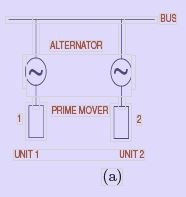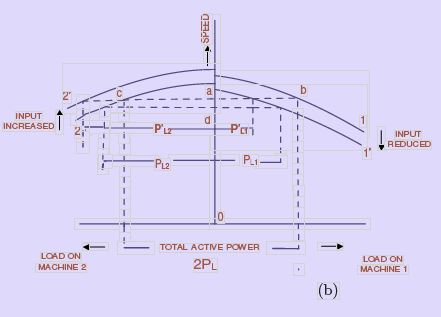Figure 37: Interconnection and load sharing

The initial operating points are indicated on the characteristic by points b and c, the busbar speed (or frequency) being given by the point a. The load on each machine is PL. the total load being 2PL. To reduce the load on unit 1, its input is decreased (by reducing the throttle opening) so that the prime-mover characteristic is now given by 1′ . The total load being constant, the loads shared by the machines are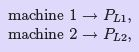the total load being PL1 + PL2 = 2PL, and the bus frequency given by the point d is reduced.
To maintain the bus frequency constant at its original value (given by point a) the input to unit 2 must be suitably increased so that its speed-load characteristic is given by 2′ . The ﬁnal load sharing is thus given by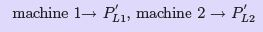and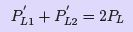(46)

Generator input and output

For any load conditions represented e.g. by Fig. 38 the output per phase is P = V I cos φ.
The electrical power converted from mechanical power input is per phase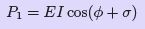(47)
Resolving E along I,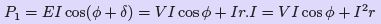(48)

The electrical input is thus the output plus the I2R loss, as might be expected. The prime mover must naturally supply also the friction, windage and core losses, which do not appear in the complexor diagram.
For a given load current I at external phase-angle φ to V , the magnitude and phase of E are determined by Zs The impedance angle θ is arc tan(x3 /r), and using Fig. 38.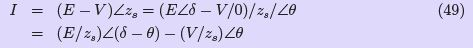when referred to the datum direction V = V ∠θ Converting to the rectangular form: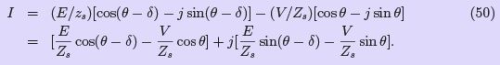These components represent I cos φ and I sin φ. The power converted internally is the sum of the corresponding components of the current with E cos δ and E sin δ, to give P1 = E cos(φ + δ):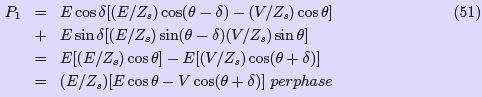The output power is V I cos φ, which is given similarly by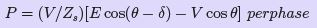(52)

In large machines the resistance is small compared with the synchronous reactance so that θ = arc tan(xs/r) ≃ 90◦.Eqn. 50 and Eqn. 52 the simplify to P1 = P , where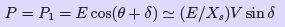(53)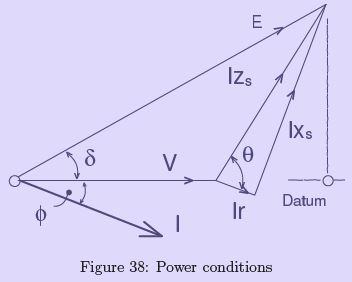Thus the power developed by a synchronous machine with given values of E , V and Z, is proportional to sin δ or, for small angles, to δ itself. The displacement angle δ represents the change in relative position between the rotor and resultant pole-axes and sis proportional to the load power. The term load-, power- or torque-angle may be applied to δ.
An obvious deduction from Eqn. 53 is that the greater the ﬁeld excitation (corresponding to E ), the greater is the output per unit angle δ; that is, the more stable will be the operation.

Synchronous Machine on Inﬁnite Bus-bars.

So far we have discussed the behavior of a synchronous generator or a pair of synchronous generator supplying a single concentrated load. In view of the tremendous increase in the size of interconnected transmission and distribution systems in the last few decades, and the power generation is contracted at a few large power stations. The generating plant capacity is of a few hundred or thousand MVAs. In such a plant several generators (of say a few hundred 100 kvAs each) will be operated in parallel. Not all of them will be operating simultaneously as we may not have the demand for the total cdapacity of the plant all the time. Assume the behaviour of a single machine connected to this type of a large generating plant is not likely to disturb the voltage and frequency provided the rating of the machine is only a fraction of the total capacity of the generating plant. In the limit,

we may presume that the generating plant maintains an invariable voltage and frequency at all points.In other words a network has zero impedance and inﬁnite rotational inertia. A synchronous machine connected to such a network is said to be operating on inﬁnite bus-bars.

As such, we can expect that, characteristics of a synchronous generator on inﬁnite bus-bars are going to be quite diﬀerent from those when it operates on its own concentrated load. As already described, a change in the excitation changes the terminal voltage, while the power factor is determined by the load, supplied by the stand alone synchronous generator. On the other hand, no alteration of the excitation can change the terminal voltage, (which is ﬁxed by the network) when it is connected to bus bars, the power factor, however, is aﬀected. In both cases the power developed by a generator depends on the mechanical power supplied. Likewise the electrical power received by a motor depends on the mechanical load applied at its shaft.

Practically all synchronous motors and generators in normal industrial use on large power supply systems can be considered as connected to inﬁnite bus-bars, the former because they are relatively small, the latter on account of the modern automatic voltage regulators for keeping the voltage practical, constant at all loads. The behaviour of the synchronous machine connected to inﬁnite bus bars can be easily described from the electrical load diagram of a synchronous generator.

Basis for drawing the general load diagram.

Consider a synchronous machine connected to inﬁnite bus bars (of constant-voltage, constantfrequency) of phase voltage V, Fig. 39. Let the machine run on no load with mechanical and core losses only supplied. If the e.m.f. E be adjusted to equality with V , no current will ﬂow into or out of the armature on account of the exact balance between the e.m.f. and the busbar voltage. This will be the case when a synchronous generator is just parallel to inﬁnite bus bar. If the excitation,If is reduced, machine E will tend to be less than V , so that a leading current Ir will ﬂow which will add to the ﬁeld ampere-turns due to direct magnetizing eﬀect of armature reaction. Under the assumption of constant synchronous impedance, this is taken into account by IZs as the diﬀerence between E and V . The current Ir must be completely reactive because the machine is on no-load and no electrical power is being supplied to or by the machine, as it is on no-load. If now the excitation be increased, E will tend to be greater than V . A current will therefore be circulated in the armature circuit, this time a lagging current which will reduce the net excitation due to the demagnetizing eﬀect of armature reaction so that the machine will again generates a voltage equal to that of the constant bus-bar voltage. The synchronous impedance drop Ir Zs is, as before, the diﬀerence between E and V , and there should be only a zero-power-factor lagging current, as the machine is running on no-load.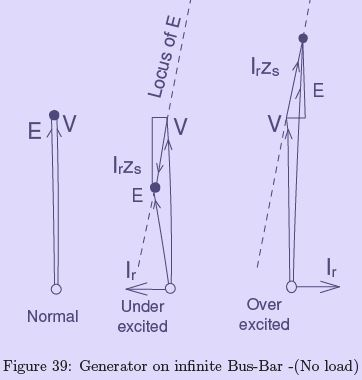Suppose the machine to be supplied with full-load mechanical power. Then as a generator it must produce the equivalent in electrical power: i.e. the output current must have an active component Iaa corresponding to full-load electrical power. For an output at exactly unity power factor, the excitation must be adjusted so that the voltage triangle E , V , IaZs, satisﬁes the conditions required, Fig. 40. If the excitation be reduced, a magnetizing reactive component is supplied in addition, i.e. a leading current Iar , which assists the ﬁeld winding to produce the necessary ﬂux. If the machine is over excited, a lagging reactive demagnetizing current component is supplied, in addition to the constant power component.

In Fig. 40 the I Zs drop has been added in components corresponding to the current components Iaa and Iar . For all three diagrams of Fig. 40, Iaa and Iaa Zs are constant, since the electrical power supplied is constant. Only the component Iaa Zs (and therefore Iar ) varies with the excitation. Thus the excitation controls only the power factor of the current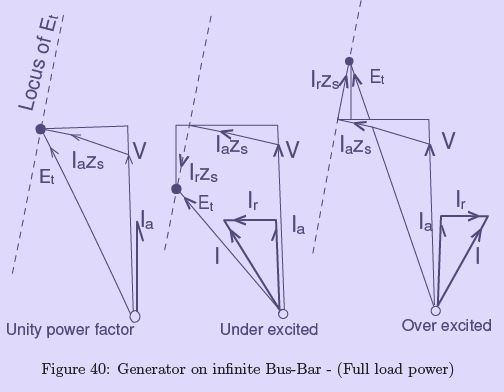supplied by the generator to the inﬁnite bus-bars and not the active power.

From this diagram for diﬀerent excitations, we can see that the extremities of the phasor of E (indicated by dots) are seen to lie on the straight line shown dashed. Since all three diagrams refer to full-load power, the dotted line becomes the locus of E and of the excitation, to scale for constant power output. This is the basis of the electrical load diagram, Fig. 42.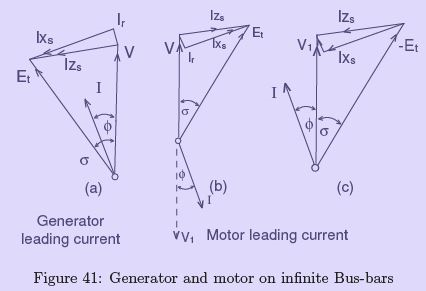A generator working-on inﬁnite bus-bars will become a motor if its excitation is maintained and the prime mover replaced by a mechanical load. The change in the phasor of Iis shown in the phasor diagrams Fig. 41(a and b). V is the output voltage of the machine, furnished by the e.m.f generated. For the motor, the current is in phase-opposition to V , since it is forced into the machine against the output voltage. For convenience, the supply voltage V1 (equal and opposite to V ) may be used when the motor is considered, and the diagram then becomes that of Fig. 41(c). The retarded angle δ of E or −E is descriptive of the fact that when the shaft of the machine is loaded, it falls slightly relative to the stator rotating ﬁeld in order to develop the torque, required by the load.

Thus, the power-angle δ, Fig. 41, plays an important role in the operation of a synchronous machine. Changes in load or excitation change its magnitude. When a machine alters from generator to motor action, δ reverses; and when δ is caused to increase exces-sively, the machine becomes unstable.

The electrical load diagram is shown in Fig. 42. The phasor V represents the constant voltage of the inﬁnite bus-bars. At the extremity of V is drawn an axis showing the direction of the Ia Zs drops—i.e. the voltage drops for unity-power-factor output currents. This axis must be drawn at the angle θ = arc tan(Xs/r) to V , to scale along the axis is a distance corresponding to, say, full load at unity power factor. At this point a line is drawn at right angles to the axis. It is the locus of the E values for constant power, or constant-electricalpower line. Other parallel lines are drawn for other loads, one through the extremity of V itself corresponding to zero power output, others on the right-hand side of V corresponding to negative power output, i.e. input to the machine as a motor.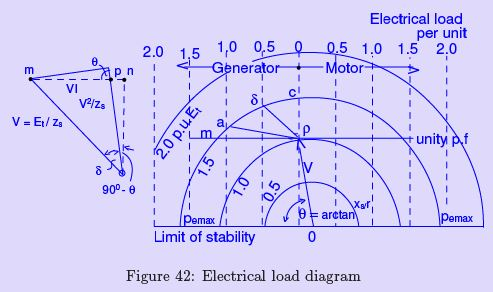The diagram solves Eqn. 52. Consider the full-load unity-power- factor case in Fig. 40, and multiply each complex voltage by the constant (V /Zs). This gives the inset in Fig. 42, from which V I = P = mp = mn − np. Now mn = (E V /Zs) sin(90 − θ + δ) and np = (V 2/Zs) cos θ, so that P is given directly by Eqn. 52.

If the excitation be ﬁxed, the extremity of the e.m.f. vector E , will have a circular locus as indicated by the circular arcs struck with O as centre. Taking 1.0 per unit E as that for which E = V on no load and no current, the per-unit excitation for any other loading condition can be found from the diagram. Thus with 1.5 per unit excitation, the machine will work on full-load power as a generator with a power factor of cos 8 lagging; on half-fun-load power with a power factor of cos 42 lagging; and on zero power output with a power-factor of zero lagging, as shown by the lines pa, pb and pc. The variation of the power output (controlled by the input from the prime mover in the case of a generator and by the load applied to the shaft for a motor) with constant excitation is thl1S accompanied by changes in the load power factor.

If the generator be provided with greater mechanical power with say, 150 per cent (or 1.5 per unit) excitation, then the output power increases with reducing power factor from lagging values until, with an output (for this case) of 1.2 per unit power (see Fig. 42), the power factor becomes unity. Thereafter the power increases with a reducing power factornow leading. Finally the excitation will not include any more constant-power lines, for the circle of its locus becomes tangential to these. If more power is supplied by the prime mover, the generator will be forced to rise out of step, and synchronous running will be lost.
The maximum power that can be generated is indicated by intercepts on the limit of stability. The typical point Pemax on the left of the load diagram is for an excitation of 1.5 per unit.

Similarly, if a motor is mechanically overloaded it will fall out of step, because of its limited electrical power intake. The point Pemax in the motor region again corresponds to 1.5 per unit excitation, and all such points again lie on the limiting-stability line. This maximum power input includes I2R loss, and the remainder-the mechanical power output-in fact becomes itself limited before maximum electrical input can be attained.

The mechanical load, or electromagnetically-converted power P1 of Eqn. 52, is for a generator the net mechanical input. For a motor it is the gross mechanical output including core friction and windage loss. A diagram resembling that of Fig. 42 could be devised* by. resolving the current along E to give P= E I cos(θ + φ). But as the terminal voltage V is taken to be constant, a new circle with another centre is needed for each value of E selected.
The following method obtains the mechanical loading from the diﬀerence I2r between P and P1.

The input to a motor is P = V1I cos φ. The electro-magnetic or converted or developed power, which includes the losses due to rotation, is P= V1 I cos φ. From the latter,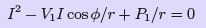(54)

giving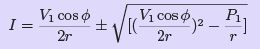(55)
For each power factor cos φ, and given voltage V1 and electro-magnetic power P1, there are two values of current, one leading and one lagging. The complexor diagrams, Fig. 43 and Fig. 45, show that there will be two corresponding values of excitation E one large and one small, associated respectively with leading and lagging reactive current components I= I sin φ. At the same time the increased I 2R loss for power factors less than unity requires the active component Ia = I cos φ to be larger. The locus of I then forms an O-curve, while the plot of the current magnitude to a base of excitation E gives a V -curve, Fig. 46.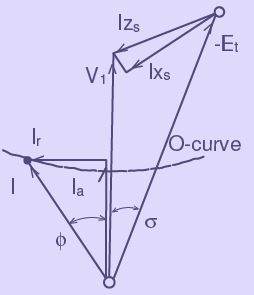Figure 43: Synchronous motor with constant output and variable excitation -Leading current

The O-curves are circular arcs, because Eqn. 55 represents the equation to a circle.
Writing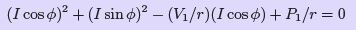(56)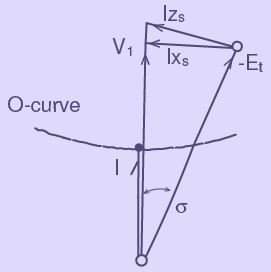Figure 44: Synchronous motor with constant output and variable excitation- Unity p.f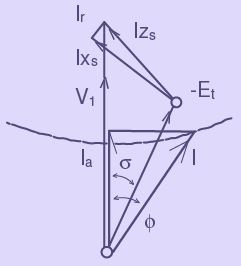Figure 45: Synchronous motor with constant output and variable excitation-Lagging current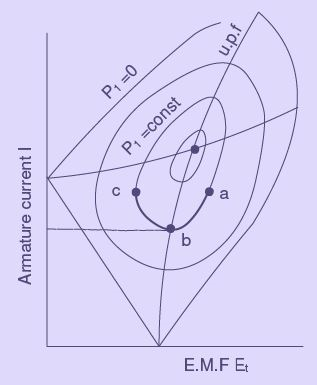Figure 46: Synchronous motor with constant output and variable excitation-V-curves

it is seen that I must lie on a circle centred at a point distant V1 /2r from the origin the axis of I cos φ, the radius of the circle being p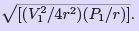The construction of the mechanical load diagram is given in Fig. 47. Let OM = V1 /2r to scale: draw with M as centre a circle of radius OM. This circle, from Eqn. 55, corresponds to P I = 0, a condition for M which the circle radius is V1/2r. The circle thus represents the current locus for zero mechanical power. Any smaller circle on centre M represents the current locus for some constant , mechanical power output P1.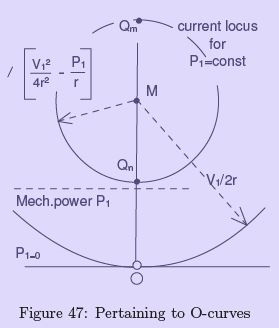For unity power factor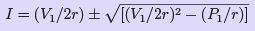(57)

Again there are in general two values O-CURVES of current for each power output P1, the smaller OQn in the working range, the greater OQm above the limit of stability.
If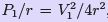, there is a single value of current I = V1/2r corresponding to the maximum power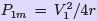. The power circle has shrunk to zero radius and becomes in fact the point M . The eﬃciency is 50 per cent, the I 2R loss being equal to the mechanical output. Such a condition is well outside the normal working range, not only because of heating but also because the stability is critical. The case corresponds to the requirement of the maximum-power-transfer theorem, commonly employed to determine maximum-poweroutput conditions in telecommunication circuits.

The completed mechanical load diagram is shown in Fig. 48, with the addition of OR = V /Zs drawn at angle arc cos(r/Zs) to OM . Circles drawn with R as centre represent constant values of E1 /Zs, or E , or the ﬁeld excitation.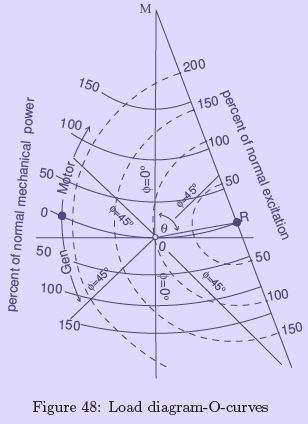O-Curves and V -Curves.

The current loci in Fig. 48 are continued below the base line for generator operation. The horizontal lines of constant mechanical power are now constant input (from the prime mover) and a departure from unity-power-factor working, giving increased currents, increases the I 2R loss and lowers the available electrical output. The whole system of lines depends, of course, on constant bus-bar voltage. The circular current loci are called the O - curves for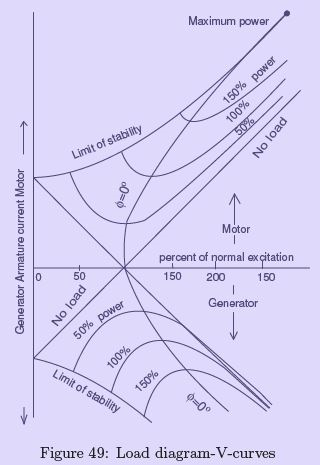constant mechanical power. Any point P on the diagram, ﬁxed by the percentage excitation and load, gives by the line OP the current to scale in magnitude and phase. Directly from the O-curves, Fig. 48, the V -curves, relating armature current and excitation for various constant mechanical loads can be derived. These are shown in Fig. 49.

Offer running on EduRev: Apply code STAYHOME200 to get INR 200 off on our premium plan EduRev Infinity!

61 docs|20 tests

,

,

,

,

,

,

,

,

,

,

,

,

,

,

,

,

,

,

,

,

,

;